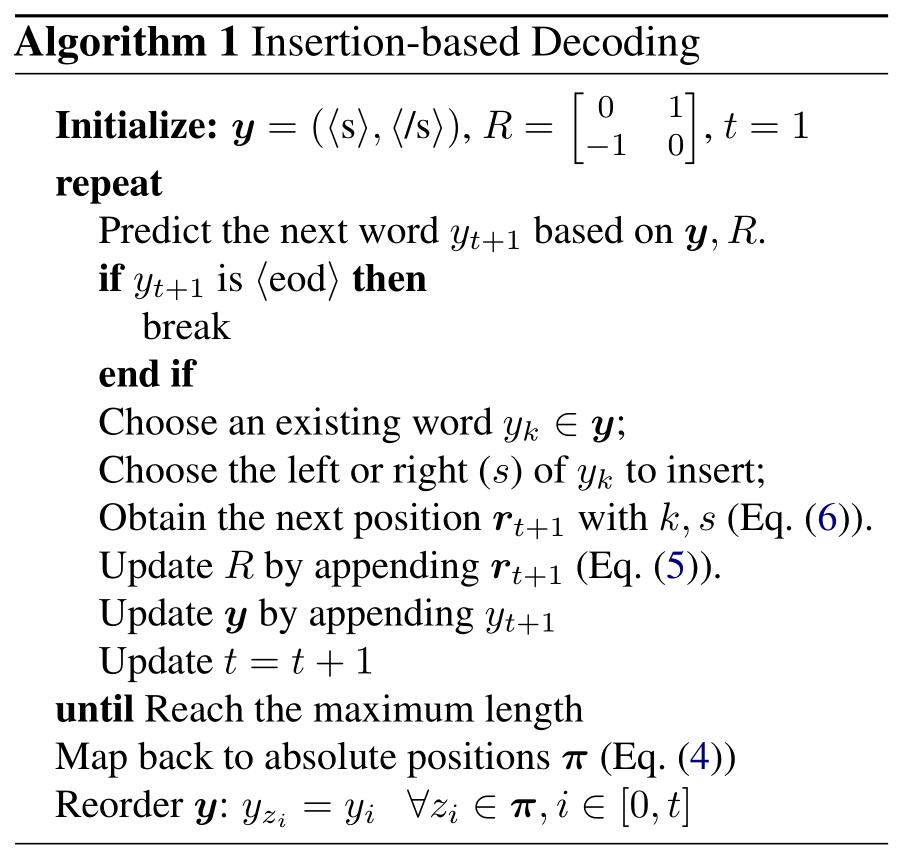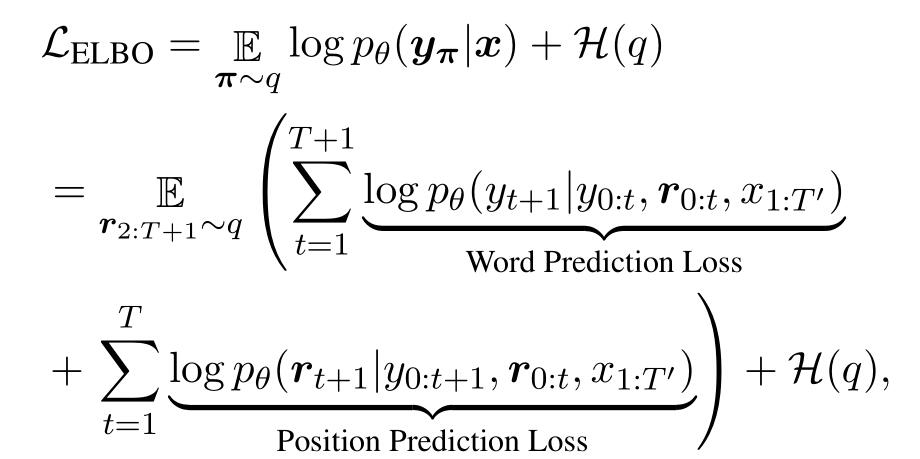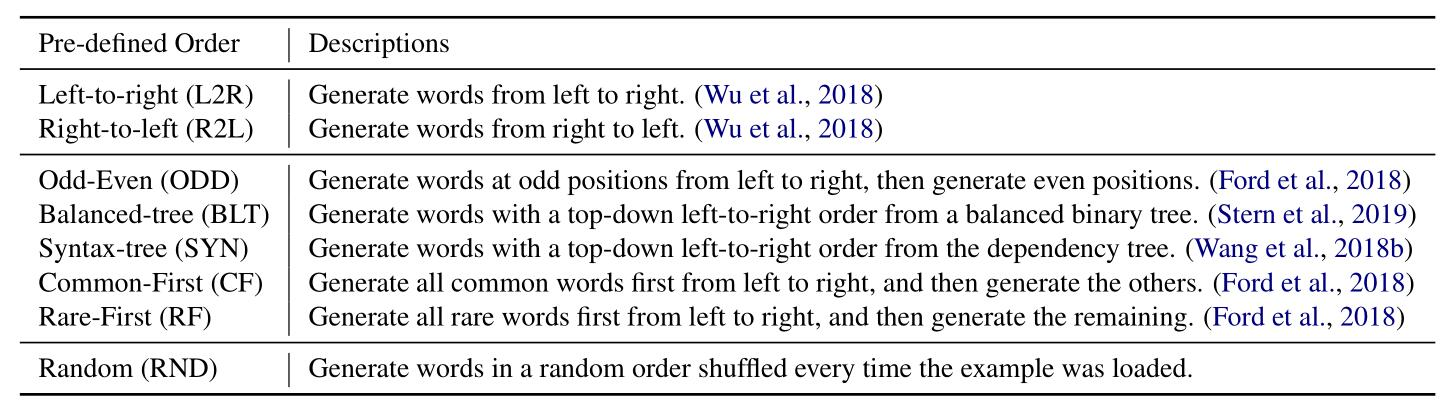# 传统序列生成模型

$p_{\theta}(y | x) = \prod_{t=0}^{T}{p_{\theta}(y_{t+1}|y_{0:t}, x_{1:T’})}$

# InDIGO

$p_{\theta}(y|x) = \sum_{\pi \in \mathcal{P}_{T}}{p_{\theta}(y_{\pi}|x)}$

$p_{\theta}(y_{\pi}|x) = p_{\theta}(y_{T+2}|y_{0:T+1}, z_{0:T+1}, x_{1:T’}) \cdot \prod_{t=1}^T{p_{\theta}(y_{t+1}, z_{t+1}|y_{0:t}, z_{0:t}, x_{1:T’})}$

$r_{i,j}$定义为：如果$w_i$绝对位置在$w_j$的左边，就取-1；如果是一个词，就取0；如果在右边，就取1。$z_i = \sum_{j}{\max\{0, r_{i, j}\}}$

# 模型

$e_{i, j} = \frac{(u_i^{\top}Q)\cdot(u_j^{\top}K + A_{[r_{i,j}+1]})^{\top}}{\sqrt{d_{\text{model}}}}$

$p(y_{t+1}, r_{t+1}|H) = p(y_{t+1}|H)\cdot p(r_{t+1}|y_{t+1}, H)$

$p_{\text{word}}(y|H) = \text{softmax}((h_t^{\top}F)\cdot W^{\top})$

$p_{\text{pointer}}(k|y_{t+1}, H) = \text{softmax}((h_t^{\top}E + W_{[y_{t+1}]})\cdot [H^{\top}C; H^{\top}D]^{\top})$

# 目标函数$\mathcal{L}_{\text{SAO}} = \frac{1}{B} \sum_{\pi \in \mathcal{B}}{\log{p_{\theta}(y_{\pi}|x)}}$

# 实验# 总结

转载规则

《论文赏析[TACL19]生成模型还在用自左向右的顺序？这篇论文教你如何自动推测最佳生成顺序》 韦阳 采用 知识共享署名 4.0 国际许可协议 进行许可。
上一篇论文赏析[EMNLP19]如何在Transformer中融入句法树信息？这里给出了一种解决方案

2020-01-06微信聊天记录深度分析，看看这一年你和谁最亲密

2019-12-29
目录